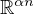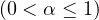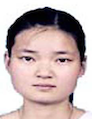Electron. J. Differential Equations, Vol. 2020 (2020), No. 72, pp. 1-20

### Representation of solutions of a second order delay differential equation Kee Qiu, Jinrong Wang

Abstract:
In this article, we study an inhomogeneous second order delay differential equation on the fractal set, based on the theory of local calculus. We introduce delay cosine and sine type matrix functions and give their properties on the fractal set. We give the representation of solutions to second order differential equations with pure delay and two delays.

Submitted March 24, 2020. Published July 7, 2020.
Math Subject Classifications: 26A33, 28A80, 34A34.
Key Words: Second order delay differential equations; delayed matrix functions; fractal set.

Show me the PDF file (355 KB), TEX file for this article.Kee Qiu School of Mathematics and Big Data Guizhou Education University Guiyang, Guizhou 550018, China email: qke456@sina.com Jinrong Wang Department of Mathematics Guizhou University Guiyang, Guizhou 550025, China email: jrwang@gzu.edu.cn

Return to the EJDE web page